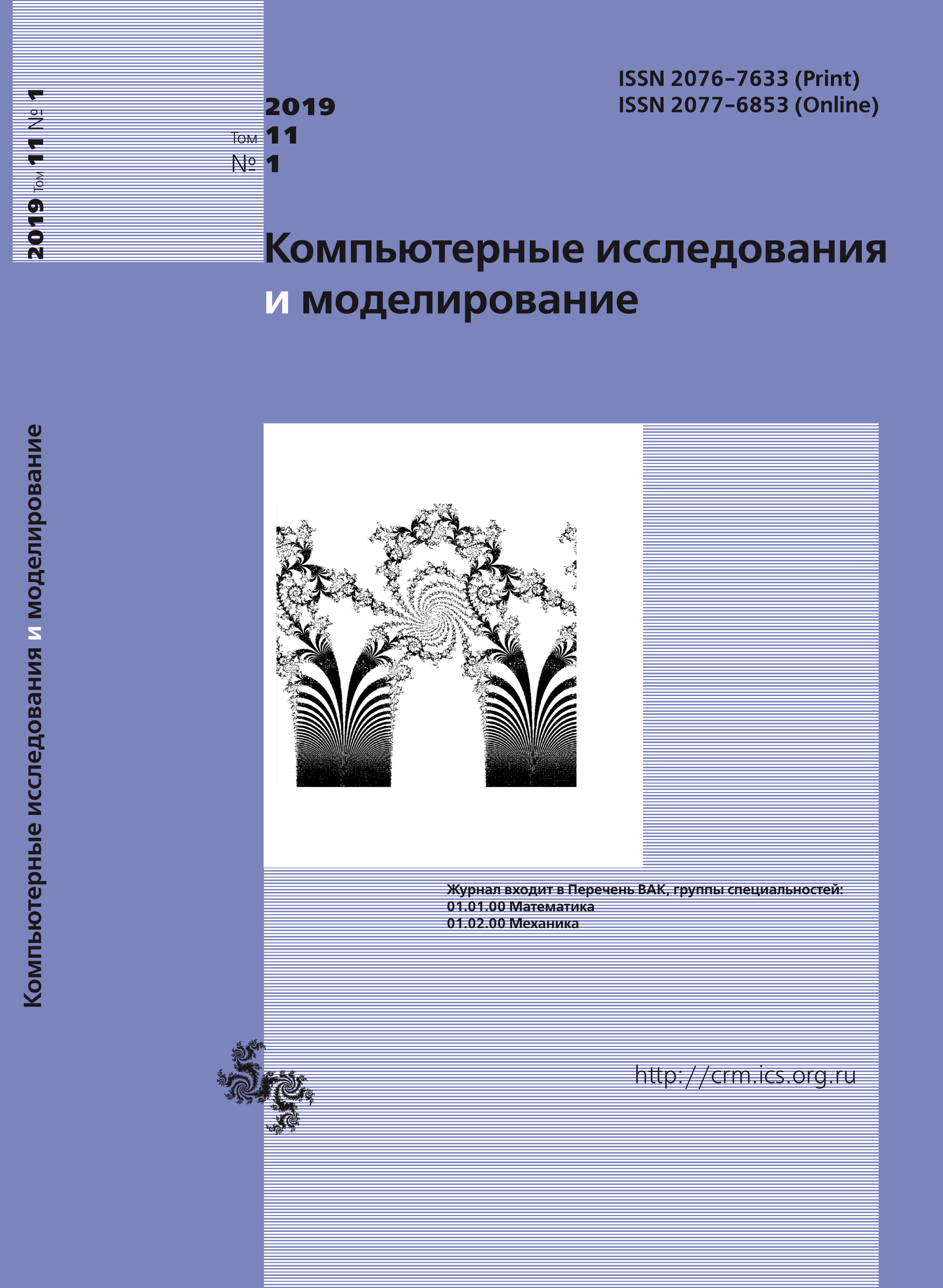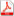Issue 1, 2019 Vol. 11

# All issues

Simulation of mixed convection of a variable viscosity fluid in a partially porous horizontal channel with a heat-generating sourcepdf (669K)  / List of references

Numerical study of unsteady mixed convection in an open partially porous horizontal channel with a heatgenerating source was performed. The outer surfaces of horizontal walls of finite thickness were adiabatic. In the channel there was a Newtonian heat-conducting fluid with a temperature-dependent viscosity. The discrete heatconducting and heat-generating source is located inside the bottom wall. The temperature of the fluid phase was equal to the temperature of the porous medium, and calculations were performed using the local thermal equilibrium model. The porous insertion is isotropic, homogeneous and permeable to fluid. The Darcy–Brinkman model was used to simulate the transport process within the porous medium. Governing equations formulated in dimensionless variables “stream function – vorticity – temperature” using the Boussinesq approximation were solved numerically by the finite difference method. The vorticity dispersion equation and energy equation were solved using locally one-dimensional Samarskii scheme. The diffusive terms were approximated by central differences, while the convective terms were approximated using monotonic Samarskii scheme. The difference equations were solved by the Thomas algorithm. The approximated Poisson equation for the stream function was solved separately by successive over-relaxation method. Optimal value of the relaxation parameter was found on the basis of computational experiments. The developed computational code was tested using a set of uniform grids and verified by comparing the results obtained of other authors.

Numerical analysis of unsteady mixed convection of variable viscosity fluid in the horizontal channel with a heat-generating source was performed for the following parameters: $\mathrm{Pr} = 7.0$, $\varepsilon = 0.8$, $\mathrm{Gr} = 10^5$, $C = 0-1$, $10^{-5} < \mathrm{Da} < 10^{-1}$, $50 < \mathrm{Re} < 500$, $\delta = l/H = 0.6-3$. Distributions of the isolines of the stream function, temperature and the dependences of the average Nusselt number and the average temperature inside the heater were obtained in a steady-state regime, when the stationary picture of the flow and heat transfer is observed. As a result we showed that an addition of a porous insertion leads to an intensification of heat removal from the surface of the energy source. The increase in the porous insertion sizes and the use of working fluid with different thermal characteristics, lead to a decrease in temperature inside the source.

Keywords: mixed convection, temperature-dependent viscosity, heat-generating source, porous medium, open channel, finite difference method
Citation in English: Astanina M.S., Sheremet M.A. Simulation of mixed convection of a variable viscosity fluid in a partially porous horizontal channel with a heat-generating source // Computer Research and Modeling, 2019, vol. 11, no. 1, pp. 95-107
Citation in English: Astanina M.S., Sheremet M.A. Simulation of mixed convection of a variable viscosity fluid in a partially porous horizontal channel with a heat-generating source // Computer Research and Modeling, 2019, vol. 11, no. 1, pp. 95-107
DOI: 10.20537/2076-7633-2019-11-1-95-107
Views (last year): 34.

Full-text version of the journal is also available on the web site of the scientific electronic library eLIBRARY.RU

The journal is included in the Russian Science Citation Index

The journal is included in the List of Russian peer-reviewed journals publishing the main research results of PhD and doctoral dissertations.

International Interdisciplinary Conference "Mathematics. Computing. Education"

The journal is included in the RSCI

Indexed in Scopus